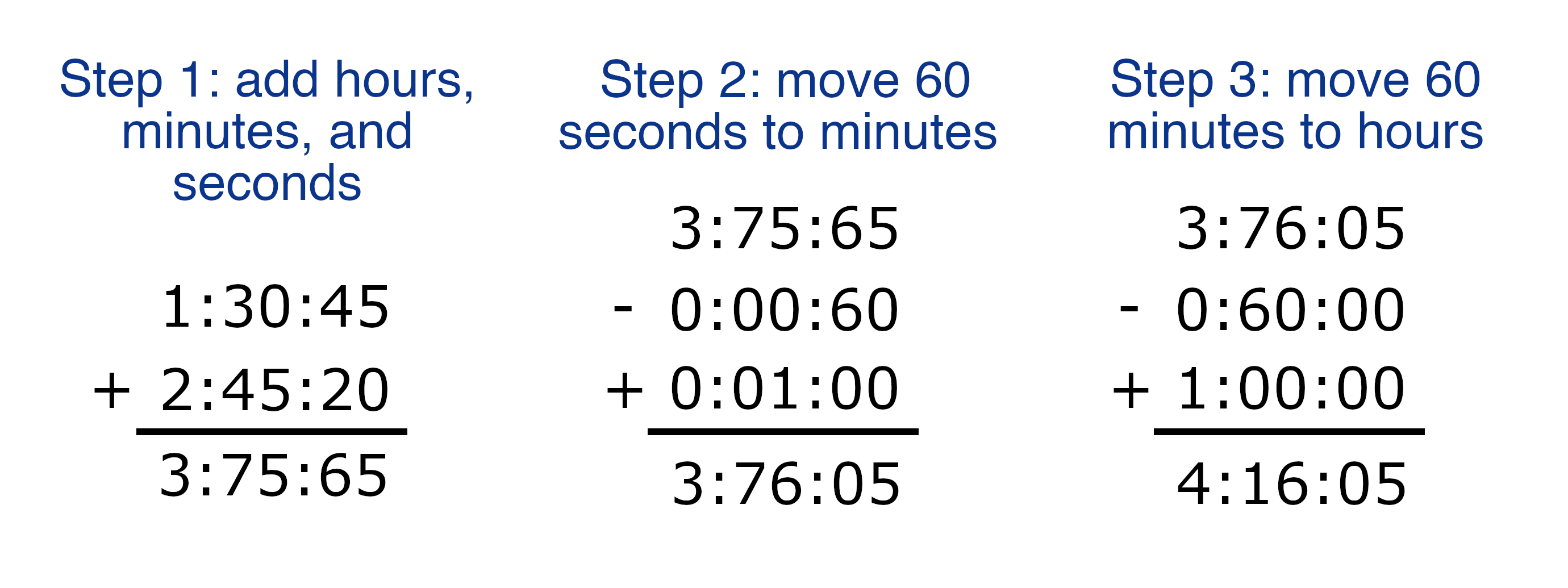Enter two times below in hours, minutes, & seconds and the time addition calculator will add them together.

Time One:

:
:
Time Two:
+
:
:

## Result:

Learn how we calculated this below

## How to Add Times Together

Adding two times or durations together might seem challenging, but it’s actually pretty easy if you follow a few simple steps.

Step One: start by adding the like components of the two times. That is, add the hours, minutes, and seconds of each time together separately.

If the resulting minutes and seconds are both less than 60, then that’s it, you’re done! If not, then proceed to step 2.

Step Two: if the resulting seconds are larger than 60, simply add 1 to the total minutes and subtract 60 from the total seconds.

Step Three: if the resulting minutes are larger than 60, then add 1 to the total hours and subtract 60 from the total minutes.

At this point you should have a final sum of the two times in hours, minutes, and seconds. Great job!

If you want an easier way to add time together and find the total hours, minutes, or seconds check out our time to decimal calculator.

For example, let’s add the times 1:30:45 and 2:45:20 together.

Add hours, minutes, and seconds separately:

hours = 1 + 2 = 3 hours
minutes = 30 + 45 = 75 minutes
seconds = 45 + 20 = 65 seconds

The resulting seconds are greater than 60, so add 1 minute and subtract 60 seconds:

minutes = 75 + 1 = 76 minutes
seconds = 65 – 60 = 5 seconds

The resulting minutes are greater than 60, so add 1 hour and subtract 60 minutes:

hours = 3 + 1 = 4 hours
minutes = 76 – 60 = 16 minutes

Thus, the sum of the times is 4:16:05, or 4 hours, 16 minutes, and 5 seconds.This calculator works great to add time; to subtract one time from another, use our subtract times calculator.